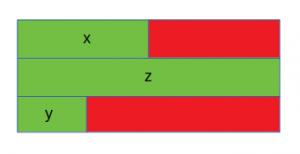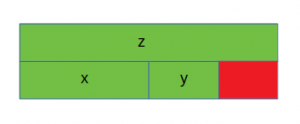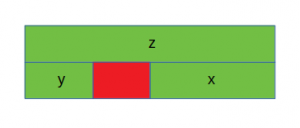# Is sizeof for a struct equal to the sum of sizeof of each member?

Prerequisite : sizeof operator in C
The sizeof for a struct is not always equal to the sum of sizeof of each individual member. This is because of the padding added by the compiler to avoid alignment issues. Padding is only added when a structure member is followed by a member with a larger size or at the end of the structure.

Different compilers might have different alignment constraints as C standards state that alignment of structure totally depends on the implementation.

Let’s take a look at the following examples for better understanding:

• Case 1:

 `// C program to illustrate ` `// size of struct ` `#include ` ` `  `int` `main() ` `{ ` ` `  `    ``struct` `A { ` ` `  `        ``// sizeof(int) = 4 ` `        ``int` `x; ` `        ``// Padding of 4 bytes ` ` `  `        ``// sizeof(double) = 8 ` `        ``double` `z; ` ` `  `        ``// sizeof(short int) = 2 ` `        ``short` `int` `y; ` `        ``// Padding of 6 bytes ` `    ``}; ` ` `  `    ``printf``(``"Size of struct: %ld"``, ``sizeof``(``struct` `A)); ` ` `  `    ``return` `0; ` `} `

Output:

```Size of struct: 24
```The red portion represents the padding added for data alignment and the green portion represents the struct members. In this case, x (int) is followed by z (double), which is larger in size as compared to x. Hence padding is added after x. Also, padding is needed at the end for data alignment.

• Case 2:

 `// C program to illustrate ` `// size of struct ` `#include ` ` `  `int` `main() ` `{ ` ` `  `    ``struct` `B { ` `        ``// sizeof(double) = 8 ` `        ``double` `z; ` ` `  `        ``// sizeof(int) = 4 ` `        ``int` `x; ` ` `  `        ``// sizeof(short int) = 2 ` `        ``short` `int` `y; ` `        ``// Padding of 2 bytes ` `    ``}; ` ` `  `    ``printf``(``"Size of struct: %ld"``, ``sizeof``(``struct` `B)); ` ` `  `    ``return` `0; ` `} `

Output:

```Size of struct: 16
```In this case, the members of the structure are sorted in decreasing order of their sizes. Hence padding is required only at the end.

• Case 3:

 `// C program to illustrate ` `// size of struct ` `#include ` ` `  `int` `main() ` `{ ` ` `  `    ``struct` `C { ` `        ``// sizeof(double) = 8 ` `        ``double` `z; ` ` `  `        ``// sizeof(short int) = 2 ` `        ``short` `int` `y; ` `        ``// Padding of 2 bytes ` ` `  `        ``// sizeof(int) = 4 ` `        ``int` `x; ` `    ``}; ` ` `  `    ``printf``(``"Size of struct: %ld"``, ``sizeof``(``struct` `C)); ` ` `  `    ``return` `0; ` `} `

Output:

```Size of struct: 16
```In this case, y (short int) is followed by x (int) and hence padding is required after y. No padding is needed at the end in this case for data alignment.

C language doesn’t allow the compilers to reorder the struct members to reduce the amount of padding. In order to minimize the amount of padding, the struct members must be sorted in a descending order (similar to the case 2).

Attention reader! Don’t stop learning now. Get hold of all the important DSA concepts with the DSA Self Paced Course at a student-friendly price and become industry ready.

My Personal Notes arrow_drop_upCheck out this Author's contributed articles.

If you like GeeksforGeeks and would like to contribute, you can also write an article using contribute.geeksforgeeks.org or mail your article to contribute@geeksforgeeks.org. See your article appearing on the GeeksforGeeks main page and help other Geeks.

Please Improve this article if you find anything incorrect by clicking on the "Improve Article" button below.

Improved By : dularnikode1

Article Tags :
Practice Tags :

15

Please write to us at contribute@geeksforgeeks.org to report any issue with the above content.